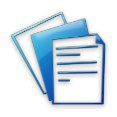#- PCLL Conversion Notes!

## Why choose our PCLL Conversion Notes?

Helping students pass PCLL Conversion Examinations since 2010

Subject Free Samples Purchase
- Notes only (HK\$680)
- Notes & 1 set of Model Exams (HK\$900)
- Notes & 2 sets of Model Exams (HK\$1100)
Each set of Model Exams for this subject contains at least 2 past paper questions and at least 2 model exam answers.
- Notes only (HK\$680)
- Notes & 1 set of Model Exams (HK\$900)
- Notes & 2 sets of Model Exams (HK\$1100)
Each set of Model Exams for this subject contains at least 2 past paper questions and at least 2 model exam answers.
- Notes only (HK\$680)
- Notes & 1 set of Model Exams (HK\$900)
- Notes & 2 sets of Model Exams (HK\$1100)
Each set of Model Exams for this subject contains at least 3 past paper questions and at least 3 model exam answers.
- Notes only (HK\$680)
- Notes & 1 set of Model Exams (HK\$900)
- Notes & 2 sets of Model Exams (HK\$1100)
Each set of Model Exams for this subject contains at least 3 past paper questions and at least 3 model exam answers.
- Notes only (HK\$680)
- Notes & 1 set of Model Exams (HK\$900)
- Notes & 2 sets of Model Exams (HK\$1100)
Each set of Model Exams for this subject contains at least 3 past paper questions and at least 3 model exam answers.
- Notes only (HK\$680)
- Notes & 1 set of Model Exams (HK\$900)
- Notes & 2 sets of Model Exams (HK\$1100)
Each set of Model Exams for this subject contains at least 4 past paper questions and at least 4 model exam answers.
- Notes only (HK\$680)
- Notes & 1 set of Model Exams (HK\$900)
- Notes & 2 sets of Model Exams (HK\$1100)
Each set of Model Exams for this subject contains at least 5 past paper questions and at least 5 model exam answers.
- Notes only (HK\$680)
- Notes & 1 set of Model Exams (HK\$900)
- Notes & 2 sets of Model Exams (HK\$1100)
Each set of Model Exams for this subject contains at least 3 past paper questions and at least 3 model exam answers.
Package A - Two subjects
- Notes & 1 set of Model Exams (HK\$1580)
Over HK\$200 discount!
- Notes & 2 sets of Model Exams (HK\$1880)
Over HK\$300 discount!
Package B - Four subjects
- Notes & 1 set of Model Exams (HK\$2980)
Over HK\$600 discount!
- Notes & 2 sets of Model Exams (HK\$3580)
Over HK\$800 discount!
Package C - Six subjects
- Notes & 1 set of Model Exams (HK\$4280)
Over HK\$1100 discount!
- Notes & 2 sets of Model Exams (HK\$5180)
Over HK\$1400 discount!
Package D - All eight subjects
- Notes & 1 set of Model Exams (HK\$5680)
Over HK\$1500 discount!
- Notes & 2 sets of Model Exams (HK\$6880)
Over HK\$1900 discount!
 We accept## What are PCLL Conversion Notes?

Our PCLL Conversion Notes will provide you with a clear and complete synthesis of the most important points you need for your exam. Our PCLL Conversion Notes adopt the short, concise and no-nonsense approach - we only tell you what you need to know to ace your exams. No more reading countless pages of the never-ending textbook just to find out one legal point.

## Why do I need PCLL Conversion Notes?

All of our PCLL Conversion Notes have been written by qualified experts and follow our unique method designed to deliver outstanding results. They follow a step-by-step checklist method, to ensure that you don't miss any important points in the exam. Remember to read our study tips for the most effective way of using our notes!

Introduce a friend to PCLL Conversion Notes and you will both get a 10% discount!